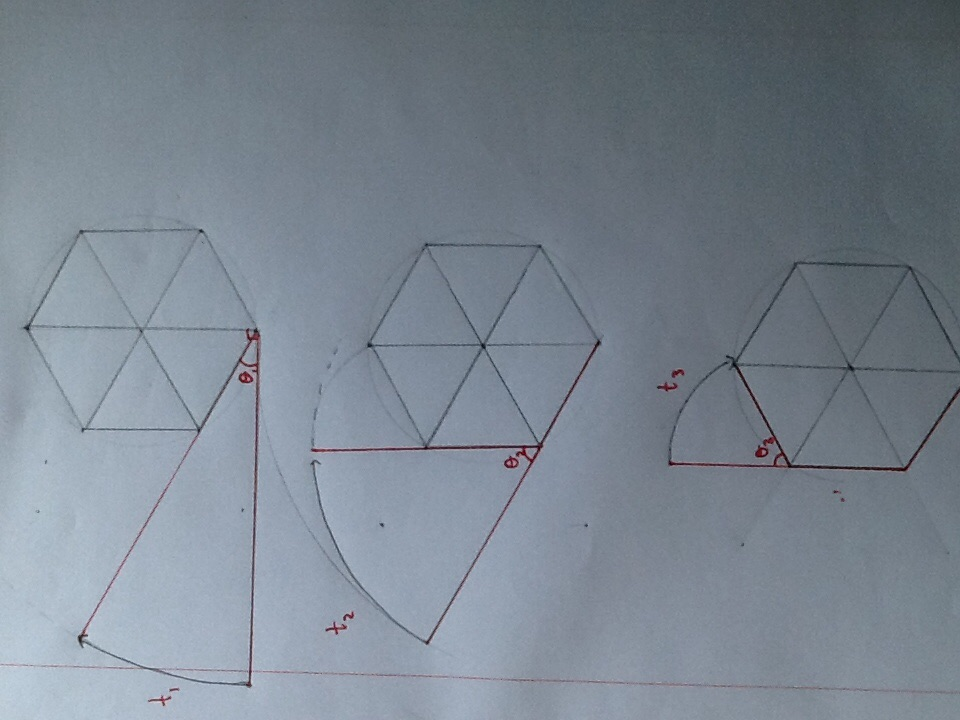# Whiplash!Consider a rope attached to one vertex of a regular hexagon, and originally resting at right angles with the symmetry line through that vertex. The rope moves towards the edge with a certain angular velocity. Once it comes in contact with the first edge, the rope bends around the first edge, and moves with the same linear velocity towards the second edge. Once it reaches the second edge, it curves around the edge and reaches the third side. Let the rope be $3$ times the side length of the hexagon.

Let the time taken to reach the first side be $t_1$. Let the time to go from the first edge to the second edge be $t_2$. Let the time to reach the third edge after reaching the second edge be $t_3$. Let the total time of motion of the rope be $T$.

Find $\frac{(t_2)(T)}{(t_1)(t_3)}$

×